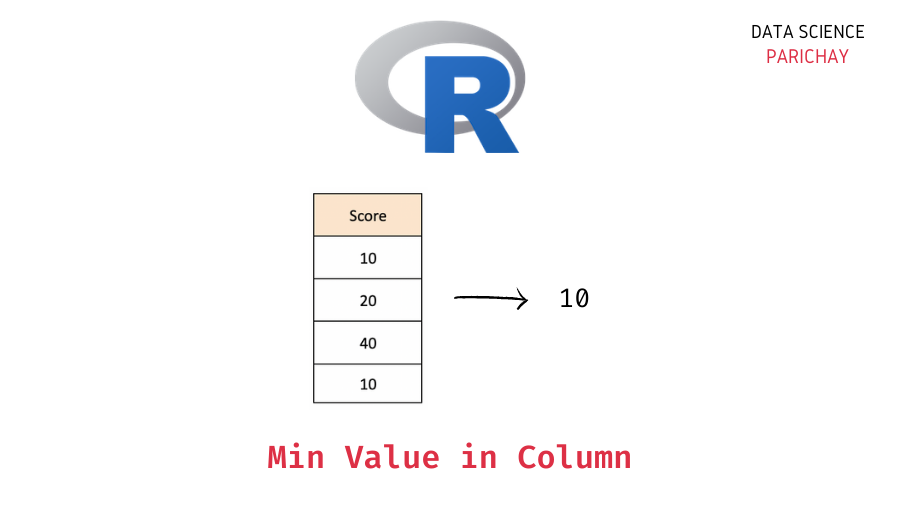# Get the Minimum Value in an R Column

The R programming language comes with a number of helpful functions to work with the data stored in data structures like vectors, lists, dataframes, etc. In this tutorial, we will look at one such function that helps us get the minimum value in a column of an R dataframe.

## How to get the min value in an R dataframe column?

You can use the built-in `min()` function in R to compute the minimum value in a dataframe column. Pass the column values as an argument to the function.

The following is the syntax –

`min(dataframe[[column_name]])`

Pass `na.rm=TRUE` to avoid the `NA` values when computing the minimum.

It returns the minimum value in the passed column.

## Steps to calculate the minimum value in an R column

Let’s now look at a step-by-step example of using the above syntax to compute the min value of a numeric column in R.

### Step 1 – Create a dataframe

First, we will create an R dataframe that we will be using throughout this tutorial.

```# create a dataframe
employees_df = data.frame(
"Name"= c("Jim", "Dwight", "Angela", "Tobi", "Kevin"),
"Age"= c(26, 28, 29, 32, 30),
"Department"= c("Sales", "Sales", "Accounting", "HR", "Accounting"),
"Salary" = c(80000, 81000, 72000, 65000, 72000)
)
# display the dataframe
print(employees_df)```

Output:

📚 Data Science Programs By Skill Level

Introductory

Intermediate ⭐⭐⭐

🔎 Find Data Science Programs 👨‍💻 111,889 already enrolled

Disclaimer: Data Science Parichay is reader supported. When you purchase a course through a link on this site, we may earn a small commission at no additional cost to you. Earned commissions help support this website and its team of writers.

```    Name Age Department Salary
1    Jim  26      Sales  80000
2 Dwight  28      Sales  81000
3 Angela  29 Accounting  72000
4   Tobi  32         HR  65000
5  Kevin  30 Accounting  72000```

We now have a dataframe containing information about some employees in an office. The dataframe has the columns – “Name”, “Age”, “Department”, and “Salary”.

### Step 2 – Calculate the minimum in a column using the `min()` function

To calculate the minimum value in a column, pass the column values as an argument to the `min()` function. You can use the `[[]]` notation to access the values of a column.

Let’s compute the minimum salary from the above data. That is, we want to know the min value in the “Salary” column.

```# min value in "Salary" column
min_salary = min(employees_df[["Salary"]])
# display the min salary
print(min_salary)```

Output:

` 65000`

The minimum value in the “Salary” column is 65000.

## Minimum value of a column with `NA` values in R

What if there are `NA` values in a column?

Let’s find out.

```# create a dataframe
employees_df = data.frame(
"Name"= c("Jim", "Dwight", "Angela", "Tobi", "Kevin"),
"Age"= c(26, 28, 29, 32, 30),
"Department"= c("Sales", "Sales", "Accounting", "HR", "Accounting"),
"Salary" = c(80000, 81000, 72000, NA, 72000)
)
# display the dataframe
print(employees_df)```

Output:

```    Name Age Department Salary
1    Jim  26      Sales  80000
2 Dwight  28      Sales  81000
3 Angela  29 Accounting  72000
4   Tobi  32         HR     NA
5  Kevin  30 Accounting  72000```

Here, we created a new dataframe such that the “Salary” column contains some `NA` values.

Now, let’s apply the `min()` function to get the minimum value in the “Salary” column.

```# min value in "Salary" column
min_salary = min(employees_df[["Salary"]])
# display the min salary
print(min_salary)```

Output:

` NA`

We get `NA` as the min value. This happened because the “Salary” column here contains `NA` values and performing any mathematical operation with `NA` results in an `NA` in R.

If you want to compute the min value in a column with `NA` values, pass `na.rm=TRUE` to the `min()` function which essentially skips these values when computing the minimum.

```# min value in "Salary" column
min_salary = min(employees_df[["Salary"]], na.rm=TRUE)
# display the min salary
print(min_salary)```

Output:

` 72000`

We now get the min value in the “Salary” column excluding the `NA` values.

## Summary – Minimum Value in an R Column

In this tutorial, we looked at how to compute the minimum value in a column of an R dataframe. The following is a short summary of the steps –

1. Create a dataframe (skip this step if you already have a dataframe on which you want to operate).
2. Use the `min()` function to compute the minimum value in the column. Pass the column vector as an argument.
3. If your column contains any NA values, pass `na.rm=TRUE` to the `min()` function to calculate the minimum excluding the `NA` values in the column.

You might also be interested in –

•